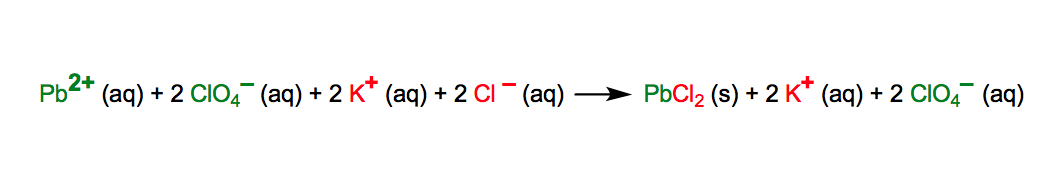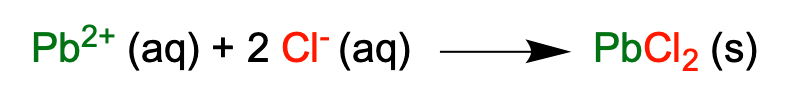Clutch Prep is now a part of Pearson
Ch.4 - Chemical Quantities & Aqueous ReactionsWorksheetSee all chapters

# Complete Ionic Equations

See all sections
Sections
Solutions
Molarity
Osmolarity
Dilutions
Solubility Rules
Electrolytes
Molecular Equations
Gas Evolution Equations
Solution Stoichiometry
Complete Ionic Equations
Calculate Oxidation Numbers
Redox Reactions
Balancing Redox Reactions: Acidic Solutions
Balancing Redox Reactions: Basic Solutions
Activity Series

Complete Ionic Equations show aqueous compounds as fully dissociated ions.

###### Complete Ionic Equations

Concept #1: Introduction to Complete Ionic Equations

The complete ionic equation shows all the aqueous compounds broken up into ions.Example #1: Convert the following balanced molecular equation into a complete ionic equation.

Concept #2: Net Ionic Equations

Net Ionic Equation shows only the ions participating in the chemical reaction, without the spectator ions.Example #2: Based on the given reactants, provide both the balanced molecular equation and the complete ionic equation.

Practice: Provide the net ionic equation that occurs when the following aqueous compounds are mixed together:

Copper (II) Bromide and Lithium Hydroxide

Practice: Which of the following reagents could be used to separate the two anions from a solution containing magnesium nitrate and cesium hydroxide?

Practice: Which of the following reagents could be used to separate the two cations from a solution containing Lead (IV) acetate and cesium permanganate?